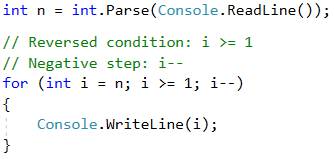# Example: Numbers N...1 in Reverse Order

Write a program that prints the numbers from n to 1 in reverse order (step of -1). For example, if n = 100, the result will be: 100, 99, 98, …, 3, 2, 1.

## Video: Numbers N...1

Watch this video lesson to learn how to print the numbers from N down to 1 (in reverse order) using a for loop: https://youtu.be/LGPZ-ug3qh0.

## Hints and Guidelines

We can solve the problem in the following way:

• We read the number n from the console input.
• We create a for loop by assigning int i = n.
• We reverse the condition of the loop: i >= 1.
• We define the size of the step: -1.
• In the body of the loop, we print the value of the current step.